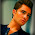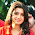### C Graph implementation with adjacency list representation using structures: Data Structure Tutorial 1

This is a quick tutorial for implementing graph data structure with adjacency list representation. Once I was
looking on the web to have a simple introductory tutorial on graphs, but unfortunately couldn’t find one
simple enough. So I decided to write this.In the adjacency list representation,

è All the vertices are stored in an array of structure containing ‘data’ and ‘link’ fields.

è The linked chains represent the edges of a vertex with its neighbors.

In the figure, there are 6 vertices, represented by the array of length 6, and the chains represent the edges.

Below is another example:Let us first define the structure of a vertex in a graph.

struct vertex
{
int vertexKey;
struct edge *edgePtr;
}vertex;

The vertexKey is the data field of the vertex, and edgePtr is the edge pointer, declared next.

The graph can be declared as:

struct vertex graph;
int vertexCount=0;

Here, we are taking the maximum number of vertices as 10.

The vertexCount gives us the number of vertices in the graph at a time.

Now, let us define the structure of an edge:

struct edge
{
int vertexIndex;
struct edge *edgePtr;
}edge;

Here, the vertexIndex field stores the index of the vertex to which the original vertex in the
array is connected.

The following figure should make the structures clear:Inserting new vertex:

We have to insert a new vertex without any edges.

This can be implemented by incrementing the vertexCount and storing the vertex data in the
graph array at vertexCount position.

void InsertVertex(int vertexKey)
{
graph[vertexCount].vertexKey=vertexKey;
graph[vertexCount].edgePtr=NULL;
vertexCount++;
}

Note that the edgePtr of  the newly inserted vertex should point to NULL, i.e., it is a null
pointer.

Now lets move on to insertion of an edge. The function is given below:

void insertEdge(int vertex1, int vertex2)
{
struct edge *e,*e1,*e2;
//For first vertex
e=graph[vertex1].edgePtr;
while(e&& e->edgePtr)
{
e=e->edgePtr;
}
e1=(struct edge *)malloc(sizeof(*e1));
e1->vertexIndex=vertex2;
e1->edgePtr=NULL;
if(e)
e->edgePtr=e1;
else
graph[vertex1].edgePtr=e1;

//For second vertex
e=graph[vertex2].edgePtr;
while(e&& e->edgePtr)
{
e=e->edgePtr;
}
e2=(struct edge *)malloc(sizeof(*e2));
e2->vertexIndex=vertex1;
e2->edgePtr=NULL;
if(e)
e->edgePtr=e2;
else
graph[vertex2].edgePtr=e2;
}

For inserting an edge, we just move to the end of the linked list of the corresponding vertex,
and insert new edge there. We have to do this for both the vertices.

And finally, here is the implementation for printing the graph:

void printGraph()
{
int i;
struct edge *e;
for(i=0;i<vertexCount;i++)
{
printf("%d(%d)",i,graph[i].vertexKey);
e=graph[i].edgePtr;
while(e)
{
printf("->%d",e->vertexIndex);
e=e->edgePtr;
}
printf("\n");
}
}

To summarize, the full code is given below:

#include<stdio.h>
#include<stdlib.h>
struct edge
{
int vertexIndex;
struct edge *edgePtr;
}edge;

struct vertex
{
int vertexKey;
struct edge *edgePtr;
}vertex;

struct vertex graph;
int vertexCount=0;

void InsertVertex(int vertexKey)
{
graph[vertexCount].vertexKey=vertexKey;
graph[vertexCount].edgePtr=NULL;
vertexCount++;
}

void insertEdge(int vertex1, int vertex2)
{
struct edge *e,*e1,*e2;
e=graph[vertex1].edgePtr;
while(e&& e->edgePtr)
{
e=e->edgePtr;
}
e1=(struct edge *)malloc(sizeof(*e1));
e1->vertexIndex=vertex2;
e1->edgePtr=NULL;
if(e)
e->edgePtr=e1;
else
graph[vertex1].edgePtr=e1;

e=graph[vertex2].edgePtr;
while(e&& e->edgePtr)
{
e=e->edgePtr;
}
e2=(struct edge *)malloc(sizeof(*e2));
e2->vertexIndex=vertex1;
e2->edgePtr=NULL;
if(e)
e->edgePtr=e2;
else
graph[vertex2].edgePtr=e2;
}

void printGraph()
{
int i;
struct edge *e;
for(i=0;i<vertexCount;i++)
{
printf("%d(%d)",i,graph[i].vertexKey);
e=graph[i].edgePtr;
while(e)
{
printf("->%d",e->vertexIndex);
e=e->edgePtr;
}
printf("\n");
}
}
main()
{
InsertVertex(5);
InsertVertex(3);
InsertVertex(4);
InsertVertex(2);
InsertVertex(9);
insertEdge(0,1);
insertEdge(0,2);
insertEdge(1,3);
insertEdge(1,4);
printGraph();
return 0;
}

The ‘main’ function is for testing of the following graph:And the final output is:The data for each vertex is written in the braces.

Now, this implementation of graph is useful for almost all applications, but is not  optimum for
‘delete vertex’ operation. For this, we will have to use a linked list instead of an array for
storing the vertices. This is shown below:That completes our first tutorial on graphs. In the next tutorial, we will discuss graph
operations like ‘depth-first-search’, ‘breadh-first-search’ etc.

If you have any queries or doubts, please ask through comments.

If you like this post, please consider to buy me a coffee.

Amount:Don’t forget to subscribe this blog through email.

### Comments

1.I would like to read second part of graph tutorial(DFS,BFS).
Where I can find it?

1.Hi, thanks for your feedback. Unfourtunately, I couldn't right the 2nd part of this tutorial (will try to write it soon).. Please refer to "Fundamentals of Data Structures" by Ellis Horowitz and Sartaz Sahni for related material.

2.Thank you sir. Sir can you help me to code the adjacency list with an input file? I hope you can help me sir. I am a 1st year computer science.

1.Hi brylle,
Here in the main() function, I have hard-coded the edges and vertices, which can be scanned using scanf(). Also, if you intend to read input from a file, you can put the scan statements in the main function, compile the program to exe, say out.exe (considering you are using windows), and in the command prompt, type "out.exe <input.txt" (without quotes), where input.txt contains the required input.

3.suppose i have 2d file
first collumn of file represent vertex of graph
n all the elements of row(except 1st )represents the edges of vertex (1st element of that row)
so how will i use this file in above code???
its very difficlut to put all the edge like u did...
can u post the code in c.

1.Hi Sidharth,
You will have to write code to read the file character by character and parse accordingly.
The pseudocode may be like this:

for(every character c in first line)
{
InsertVertex(c);
}

for(every row r except first)
{
i1=1st integer in row r;
i2=2nd integer in row r;
insertEdge(i1,i2);
}

4.Sir can you please tell me how to Compare if the graph can be represented better by using.... an adjacency matrix ... or... linked list of connected vertices for each vertex(or node) in the graph.

1.Hema,
An adjacency matrix uses O(n*n) memory. It has fast lookups to check for presence or absence of a specific edge, but slow to iterate over all edges.

Adjacency lists use memory in proportion to the number edges, which might save a lot of memory if the adjacency matrix is sparse. It is fast to iterate over all edges, but finding the presence or absence specific edge is slightly slower than with the matrix.

You might find this useful: http://stackoverflow.com/questions/2218322/what-is-better-adjacency-lists-or-adjacency-matrices-for-graph-problems-in-c

5.This comment has been removed by the author.

6.Sir I didn't get why we used first and second vertex in insertedge function.. i mean once we have added the first vertex why are we adding another in the same function!

1.I will leave it to you to figure out. (Use paper pencil and try to visualize).